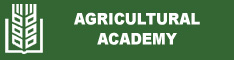Mathematical model of drying process and subsequent cooled seeds in drying-cooling unit
N. A. Assankulov
Abstract: The investigation resulted in the mathematical model of drying process and subsequent cooling of seeds in the drying-cooling unit in a system of nonlinear differential equations in ordinary and partial derivatives. The system of differential equations is reduced to a system of integral equations and transfer functions. An integral equation describes the distribution of temperature of drying agent in the office in time. The results are applied to the synthesis system to stabilize process parameters of heat treatment of seeds in the drying-cooling unit.
Keywords: a mathematical model; cooling; cooling unit; drying; seeds; the transfer function
Date published: 2019-10-03